# Gravitation

Jump to: navigation, search

Gravitation is a scientific theory which attempts to define the tendency for every object with mass to attract every other object with mass. Gravitation is what gives everyday objects weight, and what causes objects that are dropped from a height to fall to the ground. Gravitation also keeps the moon in orbit around the Earth and the Earth in orbit around the sun.

The magnitude of the force of attraction depends on the mass of the objects and the distance between them. For example, the gravitational force between two persons is minuscule; however, the gravitational force between a person and the earth is quite significant. If the person gets in a spacecraft and flies halfway to the moon, the gravitational force between him and the earth will significantly decrease because they are farther apart. The force will never become zero no matter how far he goes from the earth; however, if he goes far enough away, it will be counterbalanced by other masses' gravitational force.

The mechanism with which gravity interacts with objects has never been directly observed. Isaac Newton famously said about gravity, "Hypotheses non fingo," or, "I make no hypotheses" about how it works. More recently, Albert Einstein hypothesized as part of general relativity that gravity is a force caused by masses distorting spacetime itself. Quantum mechanics, on the other hand, hypothesizes that gravity is transmitted by a hypothetical particle, the graviton, similar to how the electromagnetic force is transmitted by the photon. The debate continues as scientists try to reconcile general relativity with quantum mechanics.

## Scientific theories of gravitation

Over the past four centuries, many scientific theories of gravitation have been proposed. Three of these theories have gained sufficient widespread acceptance to be considered, for a time, "correct" theories.

### Galilean gravitation

Gravitation has been the subject of modern scientific study for about 400 years, beginning with the work of Galileo Galilei in the late 16th century. According to a biography written by his pupil, Vincenzo Viviani, Galileo dropped objects from the Leaning Tower of Pisa to demonstrate that objects fall at the same rate regardless of mass. (Historians now disagree as to whether this experiment actually took place in the way Viviani described.) These results were in contradiction to the ancient Aristotelian conception of gravity, which stated that the speed of an object's fall was proportional to its weight.

Galileo also described a mathematical formula for the distance an object will fall in a given time, though his description was geometric rather than algebraic. His formula, that the distance fallen is proportional to the square of the elapsed time, or d ∝ t2, remains valid for many approximations today. However, Galileo's theory that the acceleration of gravity is independent on an object's height above the ground could not stand the test of time.

### Newtonian gravitation

In 1687, Sir Isaac Newton published his Principia Mathematica, an exhaustive work that laid the foundation for what we know today as classical mechanics. In this work, Newton advanced the law of universal gravitation that had been previously published by Hooke and others. Whereas Galileo described gravitation in terms of motion, Newton described it in terms of a force. Gravitation itself went from the natural tendency of objects to fall to a vector field theory in which the force between two objects was inversely proportional to the square of the distance between them.

Newton's vector equation for gravitation states that each particle in the universe attracts another particle with a force directly proportional to the product of their masses and inversely proportional to the square of the distance between them, or:Where:

•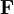is the gravitational force vector.
•and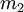are the object's masses.
•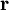is the vector that separates the objects' centers of mass.
•is the unit vector of; defined as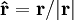•is the gravitational constant: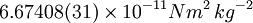Another way to express this equation, that is more convenient for programming and expansion into power series, is:Near the surface of the Earth, where the difference in gravitational acceleration at different heights is very small, Newtonian gravitation "reduces" to, or is mathematically equivalent to within a known margin of error, Galilean gravitation. That is, both Galilean gravitation and Newtonian gravitation can reasonably describe the motion of falling apples and artillery shells. But further from the surface of the Earth, the errors in Galileo's approximation become too great, and Newton's model is required.

Newton's theory of gravitation stood, refined and reformulated, but essentially unchanged, for more than two centuries.

### Relativistic gravitation

In 1915, Albert Einstein published the general theory of relativity, a radical reinterpretation of the phenomenon of gravitation, based on the work of Marcel Grossmann, David Hilbert, and others. Whereas Galileo described gravity in terms of motion, and Newton described gravity in terms of force, Einstein described gravity in terms of inertial motion in curved spacetime, such curvature being a function of the matter and fields in a given region. In the famous words of physicist John Wheeler, "Matter tells space how to curve, and space tells matter how to move."

Just as the Newtonian theory reduced to Galilean gravitation near the surface of the Earth, general relativity gravitation reduces to Newtonian gravity where gravitational fields are relatively weak. Only when gravitational fields are strong, such as the field around the sun within the orbit of Mercury, do Newton's equations fail to produce a good approximation of reality.

### The possibility of quantum gravitation

During the era when Newtonian gravity was preeminent, gravitation was considered to be one of the "fundamental forces of nature," along with electricity, magnetism and the strong and weak nuclear forces. Over the years, this interpretation has fallen out of favor, for a variety of reasons. Research into electrodynamics revealed the electric and magnetic forces to be two aspects of the same thing, and quantum mechanics described the strong and weak forces in terms of interactions mediated by bosons. Further research into quantum chromodynamics and the Standard Model increased our understanding of how electromagnetism and the nuclear forces work, but gravitation remained a thing apart.

Over the past few decades, attention has been turned toward formulating a quantum field theory of gravity which would describe the gravitational interaction in terms of a mechanism similar to the electromagnetic, strong and weak nuclear interactions.

Physicists do not universally agree that a quantum theory of gravity is inevitable, or even possible. Einstein's theory of gravitation described gravity in a way that is so fundamentally different from the other interactions in nature that many physicists no longer gravity to be a force at all. Rather, under the Einsteinian interpretation, gravitation is an inherent property of spacetime and has no analogue at the quantum level at all. Other physicists believe that the Einsteinian interpretation is merely a useful large-scale approximation of an interaction which we have not yet detected on the subatomic scale.

To date, no satisfactory theory of quantum gravity has been advanced, but research continues.